# 简单的交叉熵损失函数，你真的懂了吗？

CSDN博客：红色石头的专栏

GitHub：RedstoneWill的GitHub

L=[ylog y^+(1y)log (1y^)] L = − [ y l o g   y ^ + ( 1 − y ) l o g   ( 1 − y ^ ) ]

## 1. 交叉熵损失函数的数学原理

Sigmoid 函数的表达式和图形如下所示：

g(s)=11+es g ( s ) = 1 1 + e − s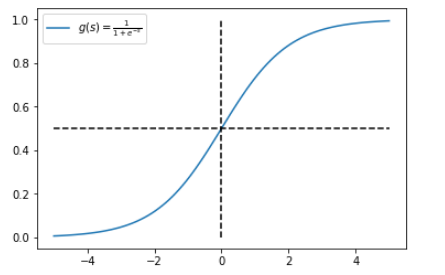y^=P(y=1|x) y ^ = P ( y = 1 | x )

1y^=P(y=0|x) 1 − y ^ = P ( y = 0 | x )

P(y|x)=y^y(1y^)1y P ( y | x ) = y ^ y ⋅ ( 1 − y ^ ) 1 − y

P(y=0|x)=1y^ P ( y = 0 | x ) = 1 − y ^

P(y=1|x)=y^ P ( y = 1 | x ) = y ^

log P(y|x)=log(y^y(1y^)1y)=ylog y^+(1y)log(1y^) l o g   P ( y | x ) = l o g ( y ^ y ⋅ ( 1 − y ^ ) 1 − y ) = y l o g   y ^ + ( 1 − y ) l o g ( 1 − y ^ )

L=[ylog y^+(1y)log (1y^)] L = − [ y l o g   y ^ + ( 1 − y ) l o g   ( 1 − y ^ ) ]

L=i=1Ny(i)log y^(i)+(1y(i))log (1y^(i)) L = ∑ i = 1 N y ( i ) l o g   y ^ ( i ) + ( 1 − y ( i ) ) l o g   ( 1 − y ^ ( i ) )

## 2. 交叉熵损失函数的直观理解

L=[ylog y^+(1y)log (1y^)] L = − [ y l o g   y ^ + ( 1 − y ) l o g   ( 1 − y ^ ) ]

L=log y^ L = − l o g   y ^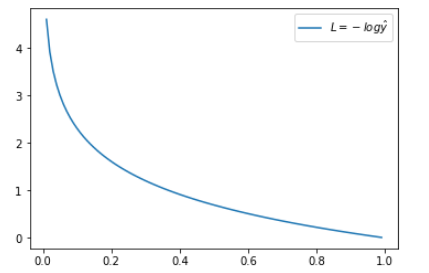L=log (1y^) L = − l o g   ( 1 − y ^ )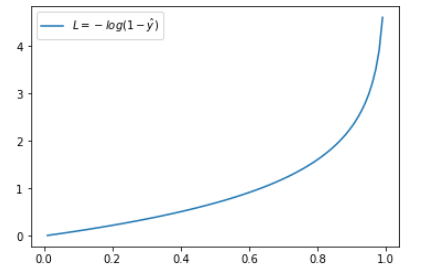## 3. 交叉熵损失函数的其它形式

1g(s)=g(s) 1 − g ( s ) = g ( − s )

P(y=+1|x)=g(s) P ( y = + 1 | x ) = g ( s )

P(y=1|x)=1g(s)=g(s) P ( y = − 1 | x ) = 1 − g ( s ) = g ( − s )

P(y|x)=g(ys) P ( y | x ) = g ( y s )

log P(y|x)=log g(ys) l o g   P ( y | x ) = l o g   g ( y s )

L=logg(ys) L = − l o g g ( y s )

L=log11+eys=log (1+eys) L = − l o g 1 1 + e − y s = l o g   ( 1 + e − y s )

L=i=1Nlog (1+eys) L = ∑ i = 1 N l o g   ( 1 + e − y s )

L=log (1+es) L = l o g   ( 1 + e − s )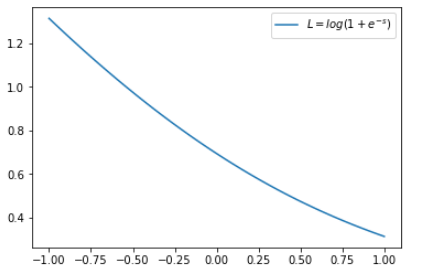L=log(1+es) L = l o g ( 1 + e s )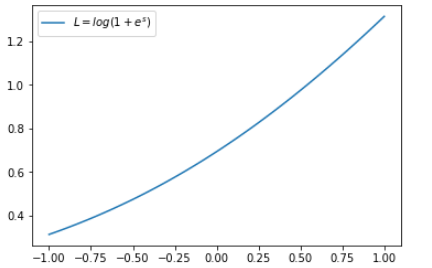## 4. 总结02-042万+

12-19341
01-024771
06-125万+
11-068290
03-301276
12-161万+
10-197627
12-07257
04-021440
07-2510万+
01-151314
02-138495
11-24852
03-27779
11-23604

### “相关推荐”对你有帮助么？

•非常没帮助
•没帮助
•一般
•有帮助
•非常有帮助¥2 ¥4 ¥6 ¥10 ¥20余额支付 (余额：-- )扫码支付获取中扫码支付点击重新获取扫码支付1.余额是钱包充值的虚拟货币，按照1:1的比例进行支付金额的抵扣。
2.余额无法直接购买下载，可以购买VIP、C币套餐、付费专栏及课程。余额充值